# Karnataka 2nd PUC Maths Important QuestionsWith Answers

For students of Maths stream, the 2nd puc Karnataka board is a very critical year, as it’s time for them to acquire more in-depth knowledge in their stream. If a student is able to pass successfully this year, then they can go for further studies to professional colleges or other, as per their preference. The marks that the students obtain in these exams are considered for admission to universities, technical and medical courses. For this, students will have to practise and learn every subject including Maths very thoroughly and the Karnataka 2nd PUC Maths Important Questions will also be a very useful tool.

To help with the preparation of these exams of such great importance, BYJU’s has compiled a list of important questions from II PU Maths textbookKarnataka board.

### Karnataka 2nd PUC Maths Important Questions With Answers

Question 1: Find the area of the triangle whose vertices are (-2, -3), (3, 2) and (-1, -8) by using the determinant method.

Solution: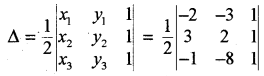= (1 / 2) (30)

= 15 square units

Question 2: Write the simplest form of tan-1 (cosx – sinx) / (cosx + sinx), 0 < x < 𝛑 / 2.

Solution:

tan-1 (cosx – sinx) / (cosx + sinx)

Divide throughout by cosx

= tan-1 [(cosx – sinx / cosx) / (cosx + sinx / cosx)]

= tan-1 [(cosx / cosx – sinx / cosx) / cosx / cosx + sinx / cosx)]

= tan-1 [(1 – tanx) / (1 + tanx)]

= tan-1 [(tan (𝛑 / 4) – tanx) / (1 + tan (𝛑 / 4) * tanx)]

= tan-1 [tan (𝛑 / 4 – x)]

= 𝛑 / 4 – x

Question 3: Find dy / dx, if x2 + xy + y2 = 100.

Solution:

x2 + xy + y2 = 100

2x + y + x * (dy / dx) + 2y * (dy / dx) = 0

(2x + y) + (dy / dx) (x + 2y) = 0

(dy / dx) (x + 2y) = – (2x + y)

(dy / dx) = – (2x + y) / (x + 2y)

Question 4: Integrate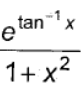with respect to x.

Solution:

Put tan-1 x = t

(1 / (1 + x2)) = dt / dx

dx = (1 + x2) dt

I = ∫et / (1 + x2) * (1 + x2) dt

= ∫et dt

= et + c

= etan^{-1} x + c

Question 5: Show that the relation R in the set A = {1, 2, 3, 4, 5} given by R = {(a, b) : |a – b| is even}, is an equivalence relation.

Solution:

R = {(1, 1), (1, 3), (1, 5), (2, 2), (2, 4), (3, 1), (3, 3), (3, 5), (4, 2), (4, 4), (5, 1), (5, 3), (5, 5)}

(a, a) ∈ R, ∀ a ∈ A

∴ R is Reflexive.

(a, b) ∈ R

⇒ (b, a) ∈ R

∴ R is symmetric.

(a, b) and (b, c) ∈ R

⇒ (a, c) ∈ R

∴ R is transitive.

∴ R is an equivalence relation.

Question 6: Find ∫x dx / (x + 1) (x + 2).

Solution:

∫x dx / (x + 1) (x + 2)

By using partial fractions method,

= A / (x + 1) + B / (x + 2)

x = A (x + 2) + B (x + 1)

Put x = – 2,

– 2 = A (- 2 + 2) + B (- 2 + 1)

– 2 = B (- 1)

B = 2

Put x = – 1,

– 1 = A (- 1 + 2) + B (- 1 + 1)

– 1 = A (1) + 0

A = – 1

∫x dx / (x + 1) (x + 2) = – 1 / (x + 1) + 2 / (x + 2)

= ∫- 1 / (x + 1) dx + 2 ∫dx / (x + 2)

= – log |x + 1| + 2 log |x + 2| + c

= – log |x + 1| + log |x + 2|2 + c

= log |(x + 2)2 / (x + 1)| + c

Question 7: Find the. area of the region bounded by the curve y = x2 and the line y = 4.

Solution: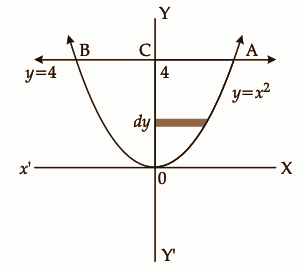The area enclosed by y = x2 and the line y = 4 is given by

Area BOAB = 2 * area of OACO

= 2 ∫04 x dy

= 2 ∫04 √y dy

= 2 [y3/2 / (3 / 2)]04

= (4 / 3) [y3/2]04

= (4 / 3) [43/2 – 03/2]

= (4 / 3) [8 – 0]

= 32 / 3 square units

Question 8: A bag contains 4 red and 4 black balls, another bag contains 2 red and 6 black balls. One of the two bags is selected at random and a ball is drawn from the bag, which is found to be red. Find the probability that the ball is drawn from the first bag.

Solution:

P (E1) = 1 / 2

P (E2) = 1 / 2

P (A / E1) = 1 / 2

P (A / E2) = 1 / 4

P (E1 / A) = [P (E1) * P (A / E1)] / [(P (E1) * P (A / E1)) + (P (E2) * P (A / E2))]

= [(1 / 2) * (1 / 2)] / [(1 / 2) * (1 / 2) + (1 / 2) * (1 / 4)]

= 2 / 3

Question 9: Sand is pouring from a pipe at a rate of 12 cubic cm l s. The falling sand forms a cone on the ground in such a way that the height of the cone is always one-sixth of the radius of the base. How fast is the height of the sand cone increasing when the height is 4 cm?

Solution:

dV / dt = 12 cm3/sec

Height of the cone = (1 / 6) of the radius of the base of the cone

Volume of the cone = (1 / 3) 𝛑r2h

= (1 / 3) 𝛑 (6h)2 h [h = r / 6]

= 12𝛑h3

dV / dt = d (12𝛑h3) / dt

12 = 12𝛑 . 3h2 (dh / dt)

1 = 𝛑 * 3 (4)2 (dh / dt)

1 / 48𝛑 = dh / dt

dh / dt = 1 / 48𝛑

= 1 / (48 * (22 / 7))

= 7 / (48 * 22)

= 0.0066 cm/sec

Question 10: Derive the equation of the line in space passing through two given points both in vector and Cartesian form.

Solution: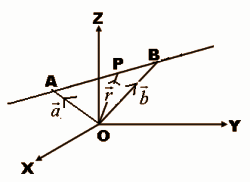Let a, b and r be the position vectors of the two points A (x1, y1, z1) is (x2, y2, z2) and p (x, y, z) respectively.

AP = OP – OA = r – a

AB = OB – OA = b – a

The point p will lie on the line AB if and only if AP and AB are collinear.

AP = ƛ AB

(r – a) = ƛ (b – a)

r = a + ƛ (b – a) is the vector equation of the line passing through two points.

Let r = xi + yj + zk, a = x1i + y1j + z1k, b = x2i + y2j + z2k, r = a + ƛ (b – a)

xi + yj + zk = x1i + y1j + z1k + ƛ ((x2 – x1) i + (y2 – y1) j + (z2 – z1) k)

= [x1 + ƛ (x2 – x1)] i + [y1 + ƛ (y2 – y1)] j + [z1 + ƛ (z2 – z1)] k

x = x1 + ƛ (x2 – x1)

x – x1 = ƛ (x2 – x1)

ƛ = (x – x1) / (x2 – x1)

y = y1 + ƛ (y2 – y1)

y – y1 = ƛ (y2 – y1)

ƛ = (y – y1) / (y2 – y1)

z = z1 + ƛ (z2 – z1)

z – z1 = ƛ (z2 – z1)

ƛ = (z – z1) / (z2 – z1)

Hence the equation of the line passing through the points (x1, y1, z1) and (x2, y2, z2) is (x – x1) / (x2 – x1) = (y – y1) / (y2 – y1) = (z – z1) / (z2 – z1).

Question 11: Solve the following system of linear equations by matrix method.

x – y + 2z = 7

3x + 4y – 5z = -5

2x – y + 3z = 12

Solution: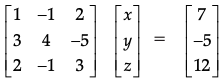AX = B

AA-1X = A-1B

IX = A-1B

X = A-1B

|A| =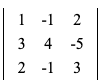= 1 · 4 · 3 + (- 1) · (- 5) · 2 + 2 · 3 · (- 1) – 2 · 4 · 2 – 1 · (- 5) · (- 1) – (- 1) · 3 · 3

= 12 + 10 – 6 – 16 – 5 + 9

= 4

Matrix of cofactors =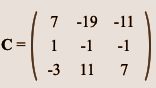Transposed matrix of cofactors = CT =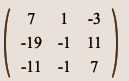A-1 = CT / |A| =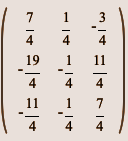X = A-1B

=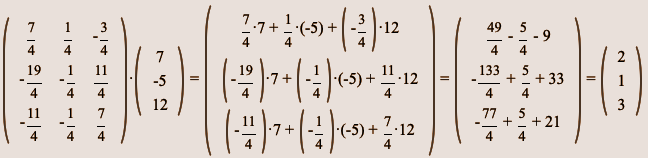x = 2, y = 1, z = 3

Question 12: Define collinear vectors.

Solution:

The two vectors are said to be collinear if they lie on the same parallel lines.

Question 13: Find the direction cosines of a line which makes equal angles with the positive coordinate axes.

Solution:

Let the direction cosines of the line make an angle α with each of the coordinate axes.

l = cos α, m = cos β, n = cos 𝝲

It is given that they make equal angles with the positive coordinate axis.

α = β = 𝝲

l2 + m2 + n2 = 1

cos2 α + cos2 β + cos2 𝝲 = 1

cos2 α + cos2 α + cos2 α = 1

3 cos2 α = 1

cos2 α = 1 / 3

cos α = √1 / 3

cos α = ± 1 / √3

The direction cosines are l = ± 1 / √3, m = ± 1 / √3, n = ± 1 / √3.

Question 14: Find the approximate change in the volume of a cube of side x metres caused by increasing the size by 3%.

Solution:

The volume of a cube (V) of side x is given by V = x3.

dV = (dV / dx) Δx

= (3x2) Δx

= (3x2) (0.03x)

= 0.09 x3

Question 15: Find the probability distribution of the number of heads in two tosses of a coin.

Solution:

When a coin is tossed twice, the number of heads maybe 0, 1, 2.

Sample space = S = {HH, HT, TH, TT}

 X 0 1 2 P (X) 1 / 4 2 / 4 1 /4

Question 16: Form the differential equation of the family of circles having a centre on y-axis and radius 3 units.

Solution:

The required equation of the circle is (x – 0)2 + (y – k)2 = 32 —- (1)

x2 + y2 + k2 – 2yk = 9 [k is any value]

On differentiating,

2x + 2y . y1 + 0 – 2k y1 = 0

x + yy1 – ky1 = 0

x + (y – k) y1 = 0

y – k = – x / y1 —- (2)

Put (2) in (1)

x2 + (-x / y1) = 9

x2 + x2 / y1 = 9

x2 (y12 + 1) = 9y12 is the required differential equation.

Question 17: If A =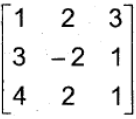then show that A3 – 23A – 40I = 0.

Solution: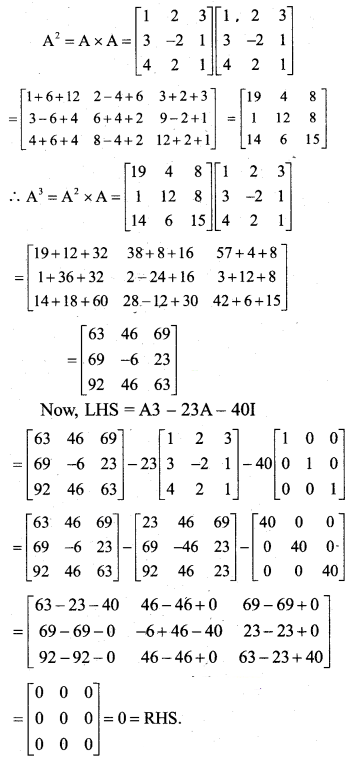Question 18: Verify Rolle’s theorem for the function f (x) = x2 + 2x – 8, x ∈ [- 4, 2].

Solution:

Since the given function is a polynomial function, it is continuous at [- 4, 2].

f ‘ (x) = 2x + 2

The given function is differentiable at [- 4, 2].

f (- 4) = 16 – 8 – 8 = 0

f (2) = 4 + 4 – 8 = 0

f (- 4) = f (2) at x ∈ [- 4, 2]

By Rolles’ theorem, there exists a real valued function c ∈ [- 4, 2]

f ‘ (c) = 0

2c + 2 = 0

2c = – 2

c = -1 ∈ [- 4, 2]

Thus Rolle’s theorem is verified.

Question 19: A ladder 5m long is leaning against a wall. The bottom of the ladder is pulled along the ground away from the wall, at a rate of 2cm/sec. How fast is its height on the wall decreasing when the foot of the ladder is 4m away from the wall?

Solution:

Let y m be the height of the wall at which the ladder touches.

Let the foot of the ladder be x m away from the wall.

By Pythagoras theorem,

x2 + y2 = 25

y = √25 – x2

The rate of change of the height (y) with respect to time (t) is given by

dy / dt = [- x / √25 – x2] (dx / dt)

It is given that dx / dt = 2cm/sec.

dy / dt = – 2x / √25 – x2

When x = 4m,

dy / dt = [- 2 * 4] / [√25 – 42]

= 8 / 3 cm/sec

Question 20: Find the two positive numbers whose sum is 15 and the sum of whose squares is minimum.

Solution:

Let the first number be x.

The sum of two numbers is 15.

⇒ First number + second number = 15

⇒ x + second number = 15

⇒ Second number = 15 – x

f (x) shows the sum of the squares of the number.

⇒ f (x) = x² + (15 – x)² = 2x² – 30x + 225

By differentiating with respect to x,

f ‘ (x) = 4x – 30

For maximum or minimum, f ‘ (x) = 0.

⇒ 4x – 30 = 0

⇒ x = 7.5

Again differentiating f ‘ (x) with respect to x,

f ” (x) = 4

At x = 7.5, f ” (x) = positive.

Thus, f (x) is minimum at x = 7.5.

Hence, the first number is 7.5 and the second number is 15 – 7.5 = 7.5.